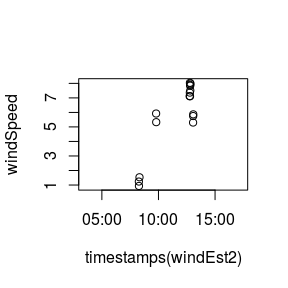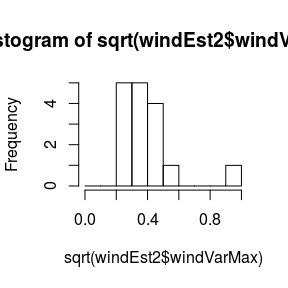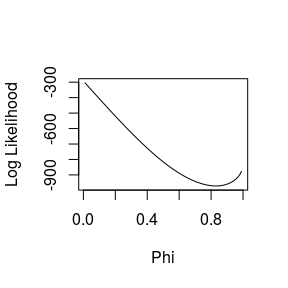# Determining parameters for wind estimation

#### 2017-02-01

This document will show how parameters used in the estimation of wind speeds can be determined from the data.

require("moveWindSpeed")

We first load an example stork track which is shipped with the moveWindSpeed package.

data = storks[]

Wind estimates are based on track segments of fixed length and fixed sampling rate. For the length of the segment we use the default value of 29:

windowSize = 29

In order to determine an appropriate sampling rate we check the sampling rate in the stork track:

tdiffs = diff(as.numeric(timestamps(data)))
quantile(tdiffs, c(0.01, 0.1, 0.5, 0.9, 0.99))
##  1% 10% 50% 90% 99%
##   1   1   1   1 900

The mayority of data points were sampled in 1 second intervals, so we choose 1 second as our sampling interval:

isSamplingRegular = 1

Wind estimation results can only be trusted if the bird is actually flying and if it is making turns, usually while circling in a thermal. We will only consider track segments in which the bird turns at least 360 degrees and has an average speed of at least 4 m/s:

isThermallingFunction = getDefaultIsThermallingFunction(360, 4)

The wind estimation is based on the assumption that, within a given track segment, the air speed of the bird fluctuates randomly around a fixed mean. For high sampling rates, however, the deviations from the mean are not statistically independent, but tend to be correlated between subsequent points. We therefore need to estimate the temporal autocorrelation paramater phi (see AR(1) processes). Phi is not estimated for each track segment separately, but across all segments of a track. For performance reasons the number of segments used in the estimation of phi can be restricted:

maxPointsToUseInEstimate = 25

With the parameters defined so far we can run the function for estimating temporal autocorrelation paramater phi:

estimationResult = estimatePhi(data, isSamplingRegular=isSamplingRegular, windowSize=windowSize, isThermallingFunction=isThermallingFunction, maxPointsToUseInEstimate=maxPointsToUseInEstimate, phiInitialEstimate=0, returnPointsUsedInEstimate=T)
## Warning in findGoodPoints(data = data, isThermallingFunction =
## isThermallingFunction, : Desired number of locations could not be found
## Warning in fn(par, ...): Location(s) omited because it fails for phi=
## 0.381966011250105 first location: 2975

The estimation function returns the estimated value of phi and the number of points used in the estimate.

estimationResult$phi ##  0.830527 estimationResult$n
##  22

In this example only 22 suitable track segments were found which is lower than the maximum specified by the parameter maxPointsToUseInEstimate and therefore triggers a warning.

The following chart shows those 22 track segments:

oo <- lapply(1:nrow(data), '+', (-(windowSize-1)/2):((windowSize-1)/2))[estimationResult$isPointUsedInEstimate] oo = oo[1:min(length(oo), maxPointsToUseInEstimate)] op = par(mfrow=c(4,6), mar=rep(0.5, 4)) # Plot track segments tmp = lapply(oo, function(ooo) plot(data[(ooo)], typ='b', xlab=NA, ylab=NA, axes=F)) par(op)We are now equipped to run the actual wind estimation along the original track. Technically wind can be estimated for every point in the track, but then the track segments used in the estimation will overlap for adjacent points. In order to avoid that we restrict the estimation to every 29th point: isFocalPoint=function(i, ts) i%%windowSize==0 Now we run the estimation function: phi = estimationResult$phi
windEst = getWindEstimates(data, isSamplingRegular=isSamplingRegular, windowSize=windowSize, isThermallingFunction=isThermallingFunction, phi=phi, isFocalPoint=isFocalPoint)
names(windEst)
##   "eobs_horizontal_accuracy_estimate"
##   "eobs_speed_accuracy_estimate"
##   "eobs_status"
##   "eobs_temperature"
##   "eobs_type_of_fix"
##   "eobs_used_time_to_get_fix"
##   "ground_speed"
##   "height_above_ellipsoid"
##  "manually_marked_outlier"
##  "event_id"
##  "estimationSuccessful"
##  "residualVarAirspeed"
##  "windX"
##  "windY"
##  "windVarX"
##  "windVarY"
##  "windCovarXY"
##  "windVarMax"
##  "airX"
##  "airY"

We only want to retain points for which the estimation was successful and where the bird was thermalling:

windEst2 = windEst[!is.na(windEst$estimationSuccessful),] Here is a simple plot of the wind speeds obtained: windSpeed = sqrt(windEst2$windX^2+windEst2$windY^2) plot(timestamps(windEst2), windSpeed)The ouput parameters windVarX, windVarY, and windCovarXY define the covariance matrix which characterizes the estimation error of the wind vector (windX, windY). The paramteter windVarMax provides a simplified error estimate, i.e. an upper bound for the error in any given direction. The following plot shows the distribution of the standard error of the wind estimate based on windVarMax hist(sqrt(windEst2$windVarMax), breaks=seq(0,1,0.1))showing that the standard error is typically in the range of 0.5 m/s.

We can also investigate how the log likelihood behaves over a series of phi values.

phis <- seq(.01, .99, by = .01)
ll <-
unlist(lapply(phis, function(x, ...) {
windEstimLogLik(getWindEstimates(phi = x, ...)$residualVarAirspeed, x) }, data = estimationResult$segments))
plot(phis, ll, type='l', xlab="Phi", ylab="Log Likelihood")